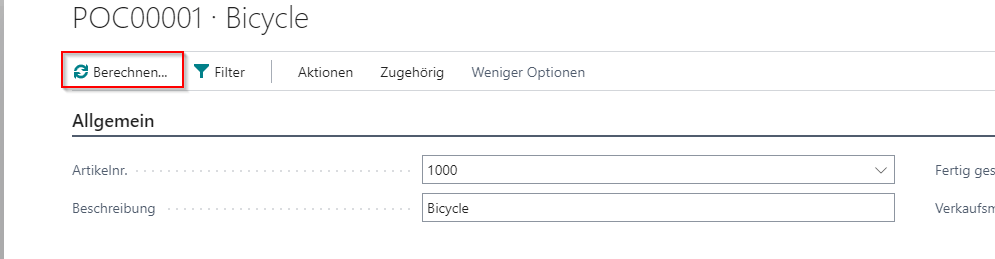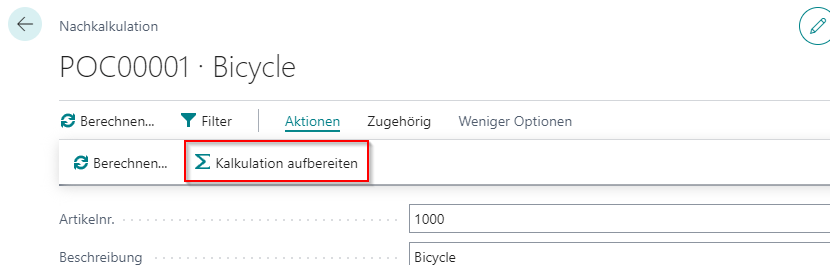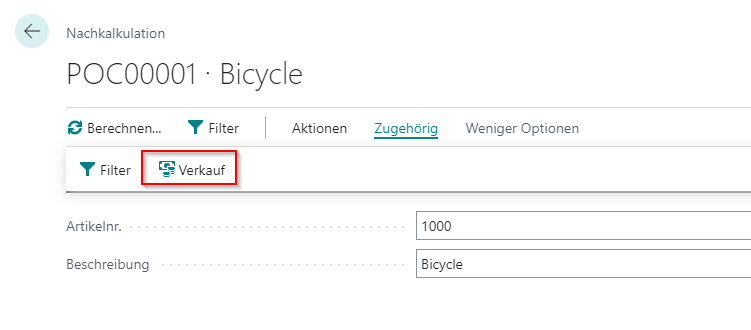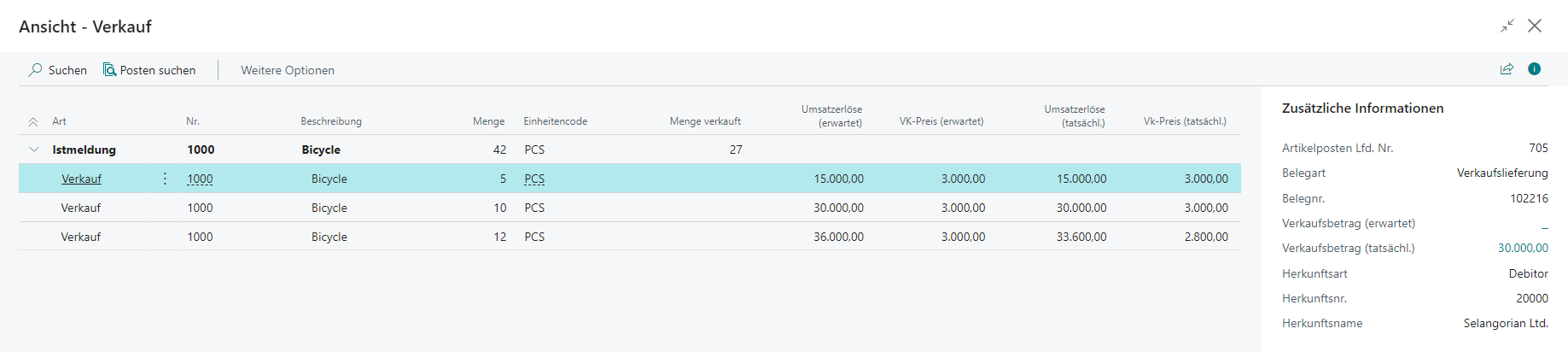1. Home
2. Docs
3. demandPlus+
4. 19*
5. Post Calculation
6. Post Calculation

# Post Calculation

The post calculation is the heart of the complete module. Here, boms and routings are broken down and costs are calculated for later evaluation.### Calculate Item

You can calculate or update the calculation using the “Calculate” button.The old lines are deleted and new ones are created. This process may also affect the associated schema.

### Filter

The post calculation searches for all “output ledger entries” for the specified item and prepares them. However, you can limit the search by specifying filters for the items.In general, all fields from the item ledger entry

### Prepare Calculation

You can prepare the calculation to apply your own schemes to the calculation.### Sales

When calculating the calculation, the system also checks what has happened to the items produced. If these have been sold, this is also logged.This will give you an overview of the documents in which the item was sold. This data will also be used later for the scheme to calculate the turnover.